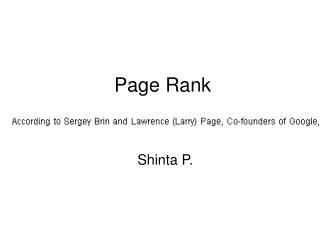DownloadDownload PresentationPage Rank

# Page Rank

Download Presentation## Page Rank

- - - - - - - - - - - - - - - - - - - - - - - - - - - E N D - - - - - - - - - - - - - - - - - - - - - - - - - - -
##### Presentation Transcript

1. Page Rank Shinta P.

2. PageRank: Random Walks Over The Web • If a user starts at a random web page and surfs by clicking links and randomly entering new URLs, what is the probability that s/he will arrive at a given page? • The PageRank of a page captures this notion • More “popular” or “worthwhile” pages get a higher rank

3. PageRank: Visually

4. PageRank: Formula Given page A, and pages T1 through Tn linking to A, PageRank is defined as: PR(A) = (1-d) + d (PR(T1)/C(T1) + ... + PR(Tn)/C(Tn)) C(P) is the cardinality (out-degree) of page P d is the damping (“random URL”) factor

5. PageRank: Intuition • Calculation is iterative: PRi+1 is based on PRi • Each page distributes its PRi to all pages it links to. Linkees add up their awarded rank fragments to find their PRi+1 • d is a tunable parameter (usually = 0.85) encapsulating the “random jump factor” PR(A) = (1-d) + d (PR(T1)/C(T1) + ... + PR(Tn)/C(Tn))

6. PageRank: Issues • Is PageRank guaranteed to converge? How quickly? • What is the “correct” value of d, and how sensitive is the algorithm to it? • What is an efficient algorithm to solve this?

7. Example: After 20 iterations